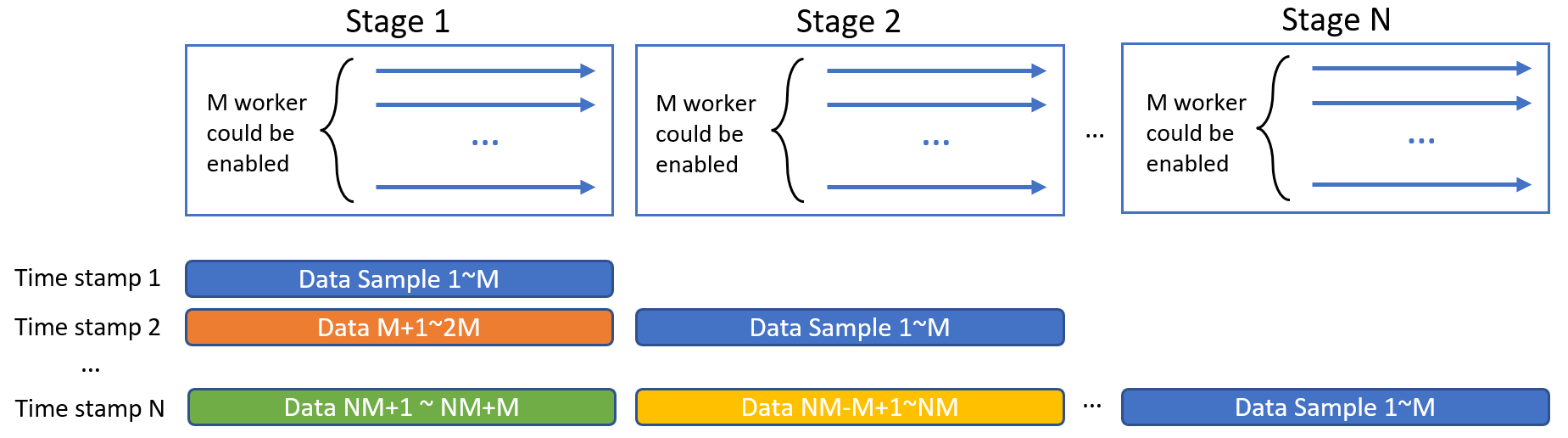View the runnable example on GitHub

# Accelerate PyTorch Inference using Async Multi-stage Pipeline#

If there are multiple stages (e.g., preprocessing, model inferencing, postprocessing, …) in your AI application, an asynchronized pipeline that parallelize different stages could significantly improve the inference throughput of the whole pipeline.

In a pipeline with N stages could work like this, where multiple (not necessarily to be the same number) workers could be enabled for each stage. The data will flow along each stages.Let’s take an ResNet-18 model pretrained on ImageNet dataset as an example. First, we load the model:

[ ]:

from torchvision.models import resnet18

model = resnet18(pretrained=True)


And we create our preprocessing function to transform image samples to tensors in our desired forms.

[ ]:

import torch
from torchvision import transforms

transform = transforms.Compose([
transforms.Resize(256),
transforms.ColorJitter(),
transforms.Resize(224),
transforms.ToTensor(),
])

def preprocess(ori_img):
img = transform(ori_img)
batch = torch.stack([img for _ in range(8)], 0)
return batch


To enable async multi-stage inference pipeline acceleration for your PyTorch model, the major work you need to make is to import BigDL-Nano Pipeline, and add each stage by specifying the stage name, the corresponding function and configs:

[ ]:

from bigdl.nano.pytorch.inference.pipeline import Pipeline

pipeline = Pipeline([
("preprocess", preprocess,  {"cores_per_worker": 4, 'worker_num': 4}),
("inference", model,  {"cores_per_worker": 4, 'worker_num': 4}),
])


📝 Note

For configs, cores_per_worker specifies the number of physical cores for each worker, default set to largest core numbers of your system. And worker_num specifies the number of workers, default set to 1.

Then you can feed your image samples to pipeline.run() API to start multi-stage pipeline inferencing.

[ ]:

import numpy as np
from PIL import Image

imarray = np.random.rand(100, 100, 3) * 255
test_image = Image.fromarray(imarray.astype('uint8')).convert('RGB')

input_samples = [test_image for _ in range(100)]
pipeline.run(input_samples)